# KSEEB Solutions for Class 7 Maths Chapter 15 Visualising Solid Shapes Ex 15.1

Students can Download Chapter 15 Visualising Solid Shapes Ex 15.1, Question and Answers, Notes Pdf, KSEEB Solutions for Class 7 Maths, Karnataka State Board Solutions help you to revise complete Syllabus and score more marks in your examinations.

## Karnataka State Syllabus Class 7 Maths Chapter 15 Visualising Solid Shapes Ex 15.1

Question 1.
Identify the nets which can be used to make cubes (cut out copies of the nets and try it):
Solution: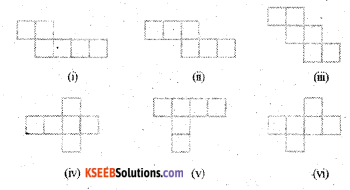In the given figures ii), iii), iv) and vi) which can be used to make cubes.Question 2.
Dice are cubes with dots on each face. Opposite faces of a die always have a total of seven dots on them.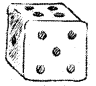Here are two nets to make dice (cubes); the numbers inserted in each square indicate the number of dots in that box.
Solution: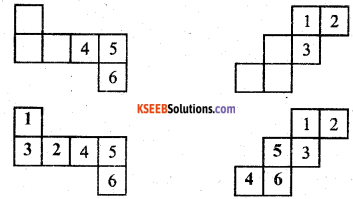Insert suitable numbers in the blanks, remembering that the number on the opposite faces should total to 7.Question 3.
Solution:
No, It is not a net for a die. Because one pair of opposite faces will have 1 and 4 on them whose total is not equal to 7 and another pair of opposite faces will have 3 and 6 on them, its total is also not equal to 7.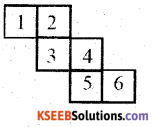Question 4.
Here is an incomplete net for making a cube. Complete it in at least two different ways. Remember that a cube has six faces. How many are there on the net here? (Give two separate diagrams. If you like, you may use a squared sheet for easy manipulation.)
Solution: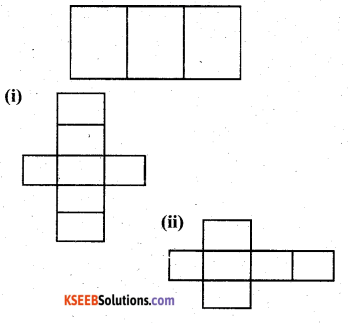In the net given here three faces are shown.

Question 5.
Match the nets with appropriate solids:Solution:
a – (ii)
b – (iii)
c – (iv)
d – (i)

error: Content is protected !!